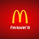TradingView
1858 views
//@version=2
// Microcana.com strategy by pilotgsms - version 4.20 <<<< Edited by Seaside420 >>>> special thanks to 55cosmicpineapple
// Hull_MA_cross added to script
strategy("Mirocana.com V4.20", overlay=true, default_qty_type=strategy.percent_of_equity, default_qty_value=100)
dt = input(defval=0.0010, title="Decision Threshold", type=float, step=0.0001)
keh=input(title="Double HullMA Cross",type=integer,defval=7, minval=1)
confidence=(security(tickerid, 'D', close)-security(tickerid, 'D', close))/security(tickerid, 'D', close)
prediction = confidence > dt ? true : confidence < -dt ? false : prediction
n2ma=2*wma(close,round(keh/2))
nma=wma(close,keh)
diff=n2ma-nma,sqn=round(sqrt(keh))
n2ma1=2*wma(close,round(keh/2))
nma1=wma(close,keh)
diff1=n2ma1-nma1,sqn1=round(sqrt(keh))
n1=wma(diff, sqn )
n2=wma(diff1, sqn )
if (prediction and n1>n2)
strategy.exit("Close", "Short")
strategy.entry("Long", strategy.long)
if (not prediction and n2>n1)
strategy.exit("Close", "Long")
strategy.entry("Short", strategy.short)
Open-source script

In true TradingView spirit, the author of this script has published it open-source, so traders can understand and verify it. Cheers to the author! You may use it for free, but reuse of this code in a publication is governed by House Rules. You can favorite it to use it on a chart.

Want to use this script on a chart?

## Commentsunfortunately this repaints the buy or sell signal on 20hrs after the event. SCAM
Reply
Profile Profile Settings Account and Billing Referred friends Coins My Support Tickets Help Center Private Messages Chat Sign Out